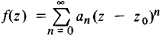# Cauchy Theorem

(redirected from Cauchy's theorem)
The following article is from The Great Soviet Encyclopedia (1979). It might be outdated or ideologically biased.

## Cauchy Theorem

a theorem concerned with the expansion of an analytic function into a power series. Suppose f(z) is a function that is single-valued and analytic in a region G, Z0 is an arbitrary (finite) point of G, and ρ is the distance from z0 to the boundary of this region. Then there exists a power series in z – z0 that converges to the function in the interior of the circle ǀz – z0ǀ = ρ:If the boundary of G reduces to the point at infinity, then ρ is infinite. This theorem was established by A. Cauchy (1831), who based it on his representation of an analytic function in the form of the so-called Cauchy integral.